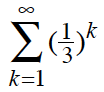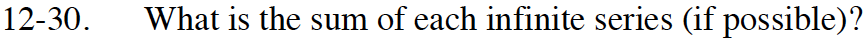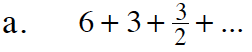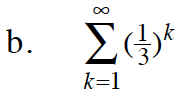### Home > INT3 > Chapter Ch12 > Lesson 12.1.2 > Problem12-30

12-30.
1. What is the sum of each infinite series (if possible)? Homework Help ✎

1. 6 + 3 ++ ...

2.How is this sequence progressing? How will that help you to find the sum?

12What are the first term and multiplier of the sequence?